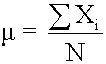Tags
Question
Population meanwhere:

• N = [...]
• Xi = [...]
• ΣXi = [...]
the number of observations in the entire population

the ith observation

add up Xi, where i is from 0 to N

Tags
Question
Population meanwhere:

• N = [...]
• Xi = [...]
• ΣXi = [...]
?

Tags
Question
Population meanwhere:

• N = [...]
• Xi = [...]
• ΣXi = [...]
the number of observations in the entire population

the ith observation

add up Xi, where i is from 0 to N
If you want to change selection, open document below and click on "Move attachment"

Subject 4. Measures of Center Tendency
represent all the observations in the data set. Population Mean The population mean is the average for a finite population. It is unique; a given population has only one mean. <span>where: N = the number of observations in the entire population X i = the ith observation ΣX i = add up X i , where i is from 0 to N Sample Mean The sample mean is the average for a sample. It is a statistic and is used to estimate the population mean.

#### Summary

status measured difficulty not learned 37% [default] 0

No repetitions